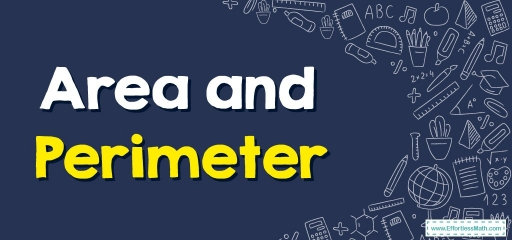# Area and Perimeter## Perimeters:

The boundary length of a closed figure is known as the perimeter of the plane figure. The perimeter units are the same as the length, such as meters, centimeters, millimeters, and more.

## Area:

Part of the plane enclosed via a simple closed figure is known as the plane region as well as the measurement of the plane region enclosed is known as the area.

The area gets measured via square units.

## Rectangles:

Rectangles are parallelograms having $$4$$ right angles. All rectangles are as well parallelograms, however, all parallelograms are not rectangles.

The perimeter $$P$$ of a rectangle is provided by the formula, $$P=2l+2w$$ where $$l$$ is the length and $$w$$ is the rectangle’s width.

The area $$A$$ of a rectangle is provided via the formula, $$A=lw$$, where $$l$$ is the length and $$w$$ is the width.

## Squares:

Squares are quadrilaterals where every side has the same length along all four corners are right angles.

The perimeter of a square is the total length of all the sides. Thus, a square’s perimeter can be determined by adding $$4$$ sides.

The perimeter of a particular square is $$a + a + a + a$$. Because all sides of a square are identical, you merely require a single side to determine the perimeter.

The perimeter of the particular square is:

$$a + a + a + a = 4a$$ units.

Thus, the formula for finding the perimeter of a square $$= 4 ×$$ (length of any $$1$$ side).

The square’s area is the product of the length of each of the sides with itself. So, $$Area = a×a$$ where $$a$$ is the length of each of the square’s sides.

Often, you’ll many times find word problems where $$2$$ of the values in $$1$$ of these formulas are provided, and you’re expected to locate the $$3$$rd.

### Area and Perimeter – Example 1:

Calculate the area of a rectangle $$10$$ cm long and $$5$$ cm wide.

Solution:

The area of a rectangle is provided via the formula, $$A=Length × width$$. Then the area of a rectangle: $$10 × 5=50$$

### Area and Perimeter – Example 2:

Sarah plans to decorate a tablecloth with a ribbon. If this tablecloth is square and each side of the square is $$3$$ meters. How much ribbon does she need to use to decorate this tablecloth?

Solution:

You know that the area of a square is equal to: $$a+a+a+a=4a$$. Then So the amount of ribbon Sarah needs is equal to: $$4 × 3=12$$ meters

## Exercises for Area and Perimeter

Salve.

1. The area of a rectangle is $$30$$ square meters. If the width of this rectangle is $$5$$ meters, what is its length?
2. What is the area of a square with $$8$$ cm on each side?
3. Find the perimeter of a rectangle with $$7$$ cm length and $$3$$ cm width.
4. What is the perimeter of a square garden with a size of $$10$$ meters on each side?

1. $$\color{blue}{6}$$
2. $$\color{blue}{64}$$
3. $$\color{blue}{20}$$
4. $$\color{blue}{40}$$

### What people say about "Area and Perimeter - Effortless Math: We Help Students Learn to LOVE Mathematics"?

No one replied yet.

X
30% OFF

Limited time only!

Save Over 30%

SAVE $5 It was$16.99 now it is \$11.99